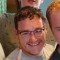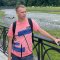# xPDOMaxValueValidationRuleLast updated Apr 12th, 2019 | Page history | Improve this page | Report an issue

## Using the Rule¶

First, our model:

``````<model package="test" baseClass="xPDOObject" platform="mysql"
defaultEngine="MyISAM" tablePrefix="test_">
<object class="myTest" table="test" extends="xPDOSimpleObject">
<field key="number" dbtype="int" precision="10"
phptype="integer" default="0" null="false" />

<validation>
<rule field="name"
name="myMaxValRule"
type="xPDOValidationRule"
rule="xPDOMaxValueValidationRule"
value="100"
message="The number cannot be greater than 100."
/>
</validation>
</object>
</model>
``````

From there, go ahead and generate the model from the XML schema. And now in a Snippet we'll call Test:

``````\$output = '';
\$obj = \$modx->newObject('myTest');
\$obj->set('number',101);
\$validator = \$obj->getValidator();
if (\$validator->validate() == false) {
\$messages = \$validator->getMessages();
foreach (\$messages as \$errorMsg) {
\$output .= \$errorMsg['message'];
}
}
``````

This will display:

The number cannot be greater than 100.